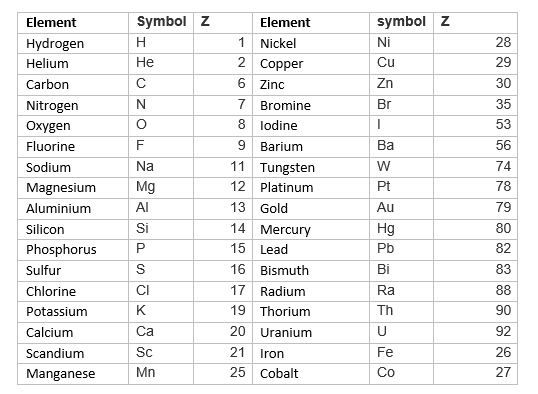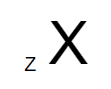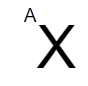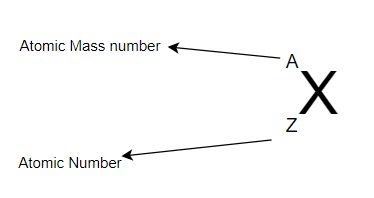# Atomic Number And Atomic Mass Number

## Atomic Number (Z)

Atomic Number of element is defined as the Number of the protons in nucleus. It is denoted by the Letter Z
Z = Number of protons present in nucleus
For example, The number of protons in hydrogen atom is 1,So Atomic Number of Hydrogen is 1
Similary the number of protons in Sodium atom is 11, So Atomic Number of Sodium is 11

Atomic Number of element depends only on the number of protons in the elements. it does not depends on number of electrons or neutron. We may use this relation when finding atomic number based on number of electrons
For neutral atom
Z = No. of protons present in nucleus = Number of Electrons

For positively Charge Atom ( Cation or +ion i,e Electron removed)
Z =No. of protons present in nucleus =Number of Electrons in charged atom + Units of Charge

For negative Charge Atom ( anion, -ion i.e Electron Added)
Z =No. of protons present in nucleus =Number of Electrons in charged atom - Units of Charge

Here is the list of atomic number of common elementsNow if the element is X, Atomic number is Z, then it is written in chemical notation asNote the subscript denote the Atomic Number

Importance of Atomic Numbers
a. Chemical properties of the element is determined by the number of electrons in the element ,which is determined by number of protons i.e atomic numbers
b. Periodic table is arranged as per the atomic number of the elements.
c. Each element has unique number of protons,So atomic number helps in identification of elements

## Atomic Mass Number(A)

Atomic Mass Number is defined as the sum of number of protons and Number of neutrons in the nucleus. It is denoted by the Letter A
A = Number of protons + Number of neutrons in nucleus
Protons and Neutrons are collectively called nucleons

It can also be written as
A = Z + Number of neutrons in nucleus
or
Number of neutrons in nucleus = A - Z

It has found that all the atomic nuclei has both the neutrons and protons ,except the most common form of hydrogen which has one proton and no neutrons.
Now if the element is X, Atomic number is A, then it is written in chemical notation asNote the superscript denote the Atomic Mass Number

Both the Atomic Number and Atomic Mass Number together is written in chemical notation asNote the superscript denote the Atomic Mass number and subscript denote the Atomic number

Atomic Mass and Atomic Mass number are different terms and should be used carefully. Atomic Mass is the mass of protons, electrons and neutrons in the atom while atomic mass number is the total number of protons and nentrons in the atom

## Solved Examples

Question 1
(i) Electron = 7, P = 7, N= 8.
(ii) e = 8, P = 9, N = 9
Find  the Charge ?
Solution
(i) no charge
(ii)  + positive

Question 2
An ion with atomic mass number 37 possess 1 unit of –ve charge. If the ion contains 11.1% mole neutrons than electrons. Find the symbol of ion.
Solution
Let the number of electrons in the ion carrying a negative charge be y.
Now according to the questions,
Number of neutrons present
= y + 11.1% of y
= y + 0.111
= 1.111 y
Number of electrons in the neutral atom = (y – 1)
Therefore, Number of protons in the neutral atom = y – 1
Now Atomic Mass = number of Neutrons + Number of protons
Therefore
37 = 1.111x + x -1
Or 2.111 x = 38
Or x = 18
Therefore no of protons = atomic no = x -1 = 18 – 1 = 17
Hence The symbol of the ion is $^{37}_{17}Cl^{-}$

Question 3
An element with mass no. 81 contains 31.7% mole neutrons as compared to protons. Assign symbol to the element.
Solution
Let the number of protons in the element be y.
Therefore,According to the question,
Number of neutrons in the element
= y + 31.7% of y
= y + 0.317 y
= 1.317 y

Mass number of the element = 81
Now we know thta,
(Number of protons + number of neutrons) = 81
y + 1.317y=81
y=35
Therefore, the number of protons in the element is  35.
Since the atomic number of an atom is defined as the number of protons present in its nucleus, the atomic number of the given element is 35.
Hence The atomic symbol of the element is  81 35 Br

Question 4
An ion with mass number 56 contains 3 unit of +ve charge & 30.4% more neutrons than e- .Assign symbol to the ion.
Solution
Let e be the number of electron
Now,As per question
Number of neutrons(n) = e + 30.4 % e
=e + 0.304 e
= 1.304 e
Now number of proton=e+3 (  As ion is positively charged)
Mass number of the element = 81
Now we know thta,
(Number of protons + number of neutrons) = 56
1.304 e + e+3=56
e=23

Therefore number of Protons =23+3=26
So, X = $^{56}_{26}Fe^{3+}$

Question 5
Calculate the number of protons, neutrons and electrons in $^{80}_{35}Br$
Solution
In this case, $^{80}_{35}Br$
Z = 35, A = 80, species is neutral
Number of protons = number of electrons
= Z = 35
Number of neutrons = A -Z = 80 - 35 = 45

Question 6
The number of electrons, protons and neutrons in a species are equal to 18, 16 and 16 respectively. Assign the proper symbol to the species.
Solution
The atomic number is equal to number of protons = 16. The element is sulphur (S).
Atomic mass number = number of protons + number of neutrons
= 16 + 16 = 32
Species is not neutral as the number of protons is not equal to electrons. It is anion (negatively charged) with charge equal to excess electrons = 18 - 16 = 2.
Symbol is $^{32}_{16}S^{2-}$